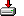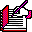#tranwrdi.sas```%macro tranwrdI(source, target, replacement);

%* Richard A. DeVenezia
%* Posted to SAS-L 1/9/03;

/* Important: RXPARSE patterns whose characters are not quoted
* are utilized in a case insensitive manner, and non-alpha
* characters when quoted are handled literally.  Thus, the
* first rxparse/change creates a case-insensitive pattern
* portion for the actual rxparse/change that follows.
* S2 and T2 are assigned 1,000 byte character values so that
* they have a valid memory space allocated to accept the
* output of rxchange.
*/

%local pattern rx times;
%local S S2 T T2;

%let S = &source;
%let T = &target;
%let T2 = %sysfunc(repeat(.,1000));
%let S2 = %sysfunc(repeat(.,1000));

%let pattern = <^'A-Za-z '>+ to '"' =1 '"' ;
%let rx = %sysfunc (rxparse ( &pattern ));
%let times = 999;
%syscall rxchange(rx, times, T, T2);
%syscall rxfree(rx);

%let pattern = &T2 TO "&replacement";
%let rx = %sysfunc (rxparse ( &pattern ));
%syscall rxchange(rx, times, S, S2);
%syscall rxfree(rx);

&S2

%mend;

/*
%put %tranwrdI (
%str
(
A string with different cases of
STRING: Like string, String, and sTRing
)
, string
, STRING
);
*/```